Stochastic Depth（随机深度网络），2016年清华的黄高在ECCV发表（妥妥的CV大佬），他后面也发表了DenseNet（2017年cv的best paper，后面有单独的博文介绍），Deep Networks with Stochastic Depth等，他在训练时对很深的ResNet做了一些压缩和剪枝，随机丢掉一些层，优化了训练速度和性能。一定程度上证明了ResNet太深了，有些层是没必要的；同时也证明了ResNet的恒等映射思想确实有效（ResNet不熟悉的同学可以看我之前的博文）。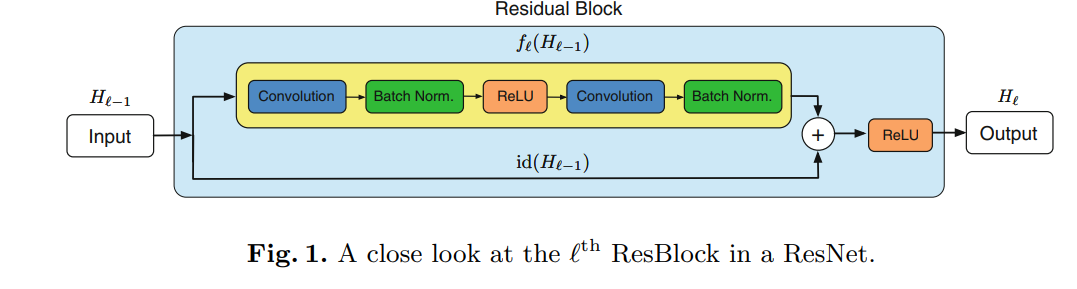Drop Path：训练时，加入了随机变量 b（伯努利随机变量），把 b*f，对整个ResBlock卷积部分做了随机丢弃。果b = 1，则简化为原始的ResNet结构；如果b = 0，则这个ResBlock未被激活，降为恒等函数。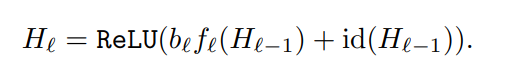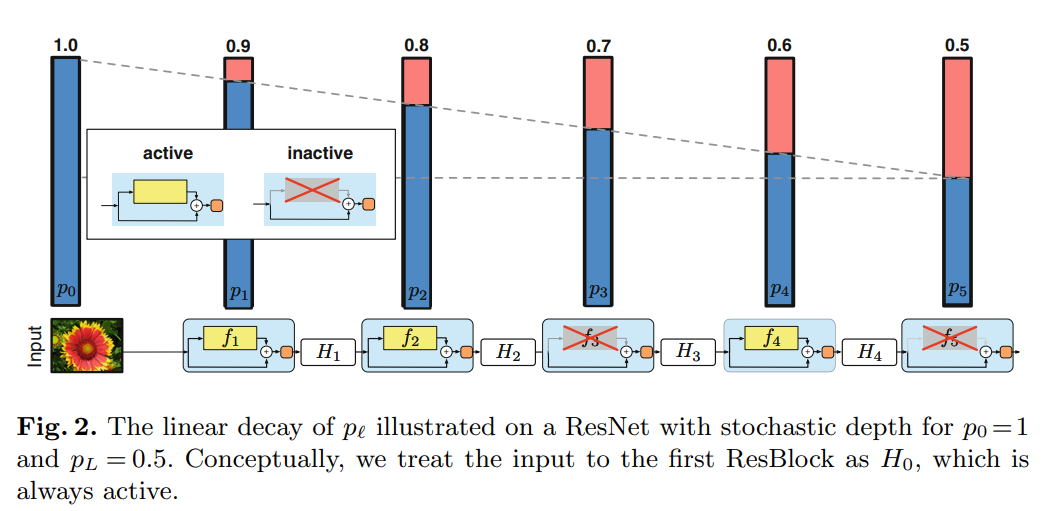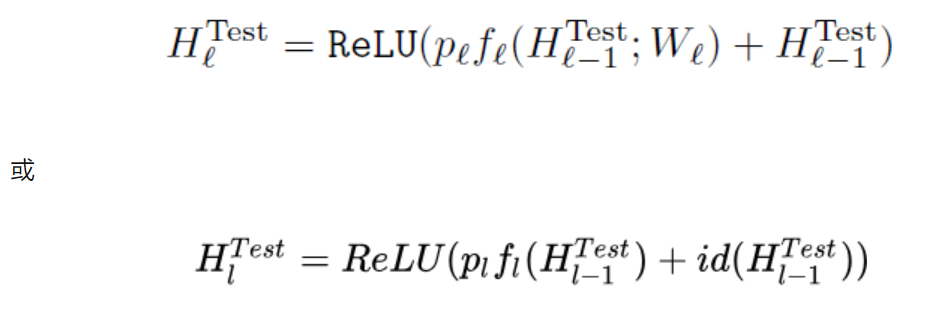ResNet的普通版和Stochastic_Depth版的比较，可见精度和速度都提高了。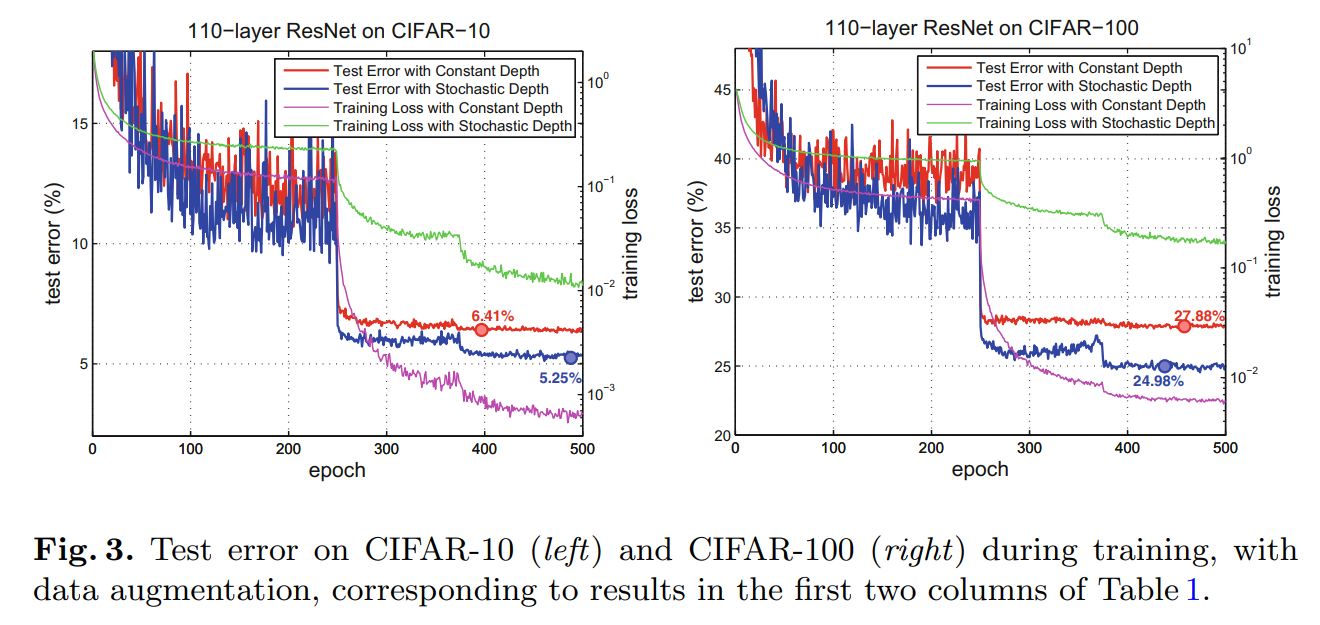def drop_path(x:Tensor, drop_probability:float=0., training:bool=False):
"""
Drop paths (Stochastic Depth) per sample (when applied in main path of residual blocks).
"Deep Networks with Stochastic Depth", https://arxiv.org/pdf/1603.09382.pdf
"""
if drop_probability == 0. or not training:
return x
survival_probability = 1 - drop_probability
shape = (x.shape,) + (1,)*(x.ndim -1) # work with diff dim tensors, not just 2D ConvNets. Output size = [batchsize, 1,1,1....]
random_tensor = survival_probability + torch.rand(shape, dtype=x.dtype, device=x.device)
random_tensor.floor()
# 假设一个神经元的输出激活值为a，在不使用dropout的情况下，其输出期望值为a，如果使用了dropout，神经元就可能有保留和关闭两种状态，
# 把它看作一个离散型随机变量，它就符合概率论中的0-1分布，其输出激活值的期望变为(1-p)*a+p*0= (1-p)a，此时若要保持期望和不使用dropout时一致，就要除以 (1-p)
output = x.div(survival_probability) * random_tensor
return output

class DropPath(nn.Module):
def __init__(self, drop_prob=None):
super().__init__()
self.drop_prob = drop_prob
def forward(self, x):
return drop_path(x, self.drop_prob, self.training)


Deep Networks with Stochastic Depth 这篇论文的创新点不在于新的网络模型，而是新的正则化方法。Drop path方法在后期的模型中被广泛应用，例如VIT, swin-Transformer等等。它与DenseNet有异曲同工之妙（后面会有专门的博文介绍），但是DenseNet太占用内存了（即便网络参数量少），这可能也是为什么DenseNet参数少，比ResNet性能好，但是，还没有ResNet流行的原因。而Drop path就可以看成一种折中的手段，因此被后续模型不断借鉴使用。